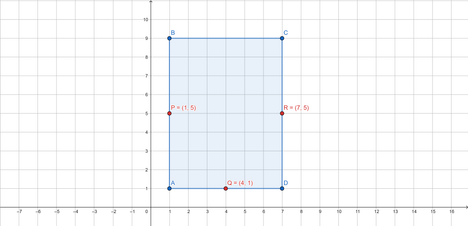# Area of ParallelogramAlbinHossainDIU Take-off Programming...
Limits 1s, 32 MB

After being rejected from all the reputed universities, MIT was their last hope. Peter, his girlfriend MJ and his best friend Ned were very excited about the letters they got from MIT. "This is our only shot.” - Ned said. "It's here or nowhere."

But alas! A fly in the ointment! MIT also rejected their application because of Spider-Man's recent controversy.

"I mean, this is so not fair!"- Peter expressed frustration. "I didn't do anything wrong. You guys definitely didn't do anything wrong."

"Expect disappointment and you will never get disappointed" - MJ consoles.

But Peter really couldn't accept it. So, He decided to meet the assistant vice chancellor of MIT to request reconsideration for the admission of MJ and Ned.

To plead the assistant vice chancellor was not an easy task. After so many requests, she agreed upon a condition. She will talk to admissions about them only if he can solve a geometry problem. The problem is very simple. Given midpoints of three sides of a parallelogram. Find the area of the parallelogram.

Peter is great at geometry. He can solve this problem too. But you know the typical super heroes! Don't have time for it. As in the meanwhile Octavius and Norman attacked on the bridge, he has to go to defend them and has thousands of lives to save. That's why, as a friend of Peter, you are asked to solve this problem.

In case you don’t remember, a parallelogram is a simple quadrilateral with two pairs of parallel sides. The opposite or facing sides of a parallelogram are of equal length and the opposite angles of a parallelogram are of equal measure. And the midpoint is the middle point of a line segment. It is equidistant from both endpoints, and it is the centroid both of the segment and of the endpoints. It bisects the segment.

## Input

The first line contains a single integer $t (1\le t \le 10^5)$ — the number of test cases. Description of the test cases follows.

Each test case contains a line containing six integers in the format $x_1 \; y_1 \; x_2 \; y_2 \; x_3 \; y_3’’$ — where $(x_1, \, y_1), \, (x_2, \, y_2)$ and $(x_3, \, y_3)$ denote the coordinates of three midpoints. All the coordinates are in between $-10^{9}$ and $10^9$ (inclusive).

## Output

For each test case print a single number — the area of the parallelogram.

## Sample

InputOutput
3
1 5 4 1 7 5
958 386 879 274 664 190
516 403 549 177 809 643

48
34888
148276


For the first test case, given three midpoints $P(1, \, 5), \, Q(4, \, 1)$ and $R(7, \, 5)$. A possible parallelogram is $ABCD$ (as shown in the figure) where $P, \, Q$ and $R$ are midpoints of sides $AB, \, AD$ and $CD$ respectively. $ABCD$ covers $48$ small square units. So, the answer for the test case is $48$.### Submit